#### 期刊菜单

Design and Implementation of Online Examination System Based on Separation of Teaching and Examination
DOI: 10.12677/SEA.2022.113069, PDF, HTML, XML, 下载: 45  浏览: 135  科研立项经费支持

Abstract: The separation of teaching and examination is conducive to the fairness and justice of teaching assessment and the scientific and effective evaluation of teaching quality. Therefore, the implementation of the separation of teaching and examination has become the trend of higher education reform. In view of the disadvantages existing in the implementation of the separation of teaching and examination, such as the lack of intelligence in the marking of subjective questions, the insufficient invigilation and the insufficient comprehensive analysis of scores, based on the consideration of technical difficulty and implementation feasibility, this paper designs and implements an online examination system based on the separation of teaching and examination, including the functions of question bank management, automatic test paper generation, online invigilation, auxiliary marking of test questions, fuzzy search of questions and automatic score analysis, which helps to alleviate the pressure of teachers, maintain the fairness of examination and improve teaching quality.

1. 引言

2. 教考分离发展现状

3. 系统需求分析与设计

3.1. 需求分析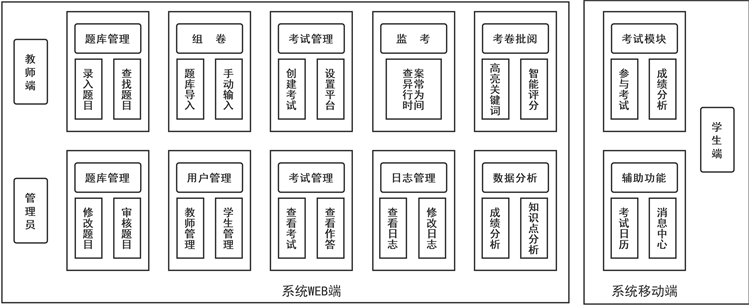Figure 1. Function module diagram of online examination system based on separation of teaching and examination

3.2. 系统架构

1) 应用层

2) 服务存储层

3) 计算处理层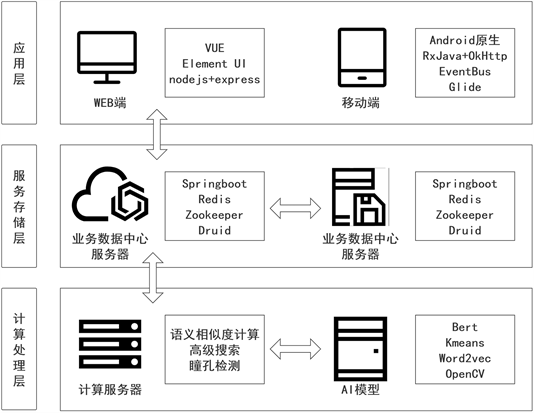Figure 2. Diagram of the system architecture

4. 系统实现

4.1. 系统WEB端

WEB端作为本系统的主要端口，为所有用户提供了对应的功能。教师登录系统后可在考试管理页面中选择添加考试，选择组卷方式后填写相应的考试名称、平台、时间、是否开启反作弊等考试信息。随后导入考生列表，查看考卷内容。试卷发布后，学生可以登录系统，选择正在进行中的考试进行答题。教师端可以监测异常情况。考试结束后，教师可以点击考试进行批改阅卷，点击自动批改按钮则可直接对客观题进行批改，并获取相应的分数。对于主观题，系统将会对答案进行提取关键字，并与学生回答关键字进行相似度分析，对学生回答进行高亮关键字效果，和计算相应的得分，以供阅卷教师进行参考，提高工作效率。系统以图表的形式，为教师提供直观的考试综合情况，可以查看考试的均分，方差等基本信息，以及考试知识点等掌握情况，如图3所示。最后系统会为教师提供合理的教学建议以供教师参考。为了提升师生的使用体验，教师在对考卷进行编辑时，可以点击导入，从已有的题库中选择所需的题目，则可以自动将题目按照题目类型导入到试卷中。同时为了提高效率，教师可以拍照上传题目，导入系统。题库管理中还为教师提供了模糊搜索的功能，方便查找试题。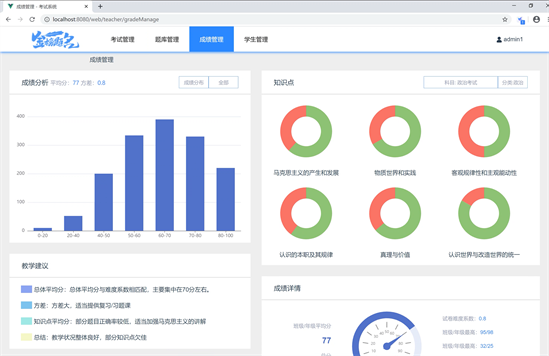Figure 3. Performance analysis

4.1.1. 试题辅助批阅

1) Sentence-Bert模型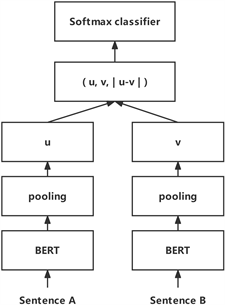Figure 4. Diagram of the Sentence Bert structure

2) 关键词算法

$t\left(u\right)=d{\sum }_{d\in adj\left[u\right]}p\left(v\to u\right)t\left(v\right)+\left(1-d\right)\frac{1}{|V|}$

3) 相似度匹配

$\begin{array}{l}W=\underset{i=1}{\overset{m}{\sum }}\underset{j=1}{\overset{n}{\sum }}{d}_{ij}{f}_{ij}\to \mathrm{min}\\ {\text{Similarity}}_{\text{key}}=\frac{1}{1+W}\end{array}$

${\text{Similarity}}_{\text{sentiment}}=\mathrm{cos}\left(\theta \right)=\frac{A\cdot B}{‖A‖‖B‖}=\frac{\underset{i=1}{\overset{n}{\sum }}{A}_{i}×{B}_{i}}{\sqrt{\underset{i=1}{\overset{n}{\sum }}{\left({A}_{i}\right)}^{2}×\underset{i=1}{\overset{n}{\sum }}{\left({B}_{i}\right)}^{2}}}$

$\text{Similarity}={\omega }_{1}\ast {\text{Similarity}}_{\text{key}}+{\omega }_{2}\ast {\text{Similarity}}_{\text{sentiment}}$

4.1.2. 题目模糊搜索

1) 题目预处理

2) 语义相似度计算

3) 输出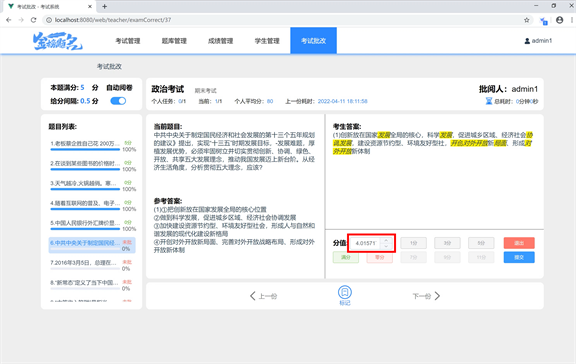Figure 5. Auxiliary marking of test questions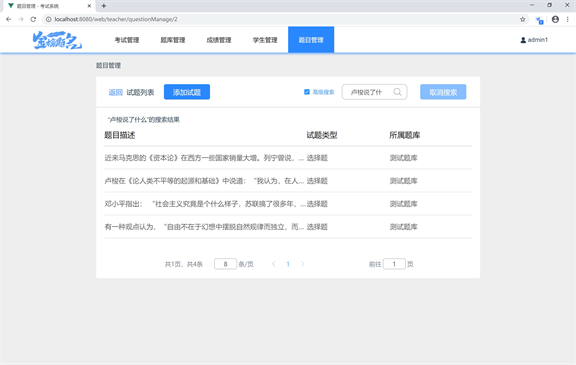Figure 6. Fuzzy search

4.2. 系统移动端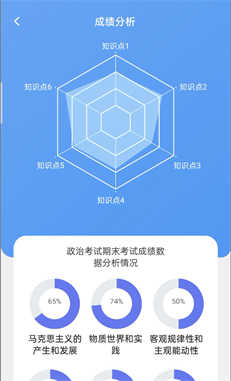Figure 7. Performance analysis (mobile terminal)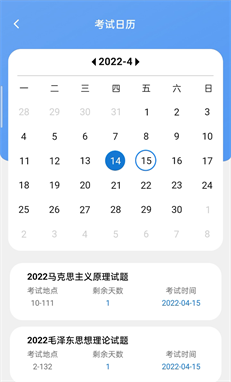Figure 8. Examination calendar

1) 调用OpenCV库识别瞳孔。

2) 按照设定的时间间隔，分别记录两个瞳孔位置。

3) 记录瞳孔位置的次数达到事先设定的阈值后，计算两个瞳孔各自的平均位置及标准差，重置记录。

4) 跳转至2)，同时开始执行异常检测。

$\begin{array}{l}\text{check}=\left(\text{pupli}-\stackrel{¯}{\text{pupli}}\right)/\sigma \left(\text{pupli}\right)\\ \text{warningoccurswhencheck}\ge \text{Parameter}\end{array}$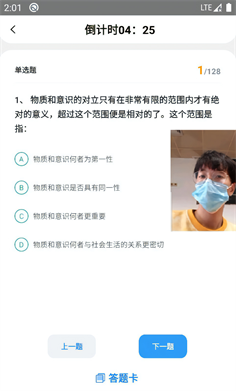Figure 9. Pupil detection-1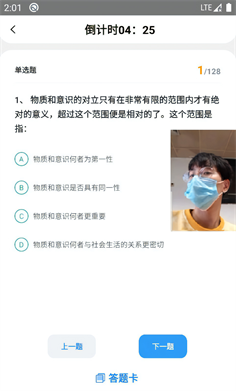Figure 10. Pupil detection-2

5. 结语

  傅军. 完善教考分离加强试题库建设与管理[J]. 考试周刊, 2007(49): 2-3.  柴春红, 刘希军. 高等数学课程“教考分离”改革的效果分析[J]. 职业时空, 2011, 7(5): 71-72.  曹晓菲, 王小才, 余柏林. 数学类课程实施教考分离模式的必要性[J]. 教育教学论坛, 2021(43): 136-139.  董杨琴, 曹洪其. 以改革课程考核为切入点推进高职院校质量工程建设[J]. 南通职业大学学报, 2010, 24(2): 59-61.  魏首柳, 柯小玲. 基于教考分离的课程试题库建设的探讨与实践[J]. 高教学刊, 2021(3): 100-103.  王玲玲. 药学《仪器分析》课程“教考分离”考核模式初探[J]. 广东化工, 2021, 48(15): 247+254.  龚晓燕, 杨浩. 基于随机搜索逐步优化的自动组卷算法及试题库结构的研究[J]. 考试周刊, 2007(49): 1-2.  蔡永茂, 汤滨, 朱科钤. 对高校教考分离考试模式的思考[J]. 教育教学论坛, 2020(42): 320-321.  乔亚男, 胡名凯, 薄钧戈. 基于特征融合的主观题智能阅卷算法研究[J]. 电气电子教学学报, 2021, 43(6): 104-111.  夏天. 词向量聚类加权TextRank的关键词抽取[J]. 数据分析与知识发现, 2017, 1(2): 28-34.